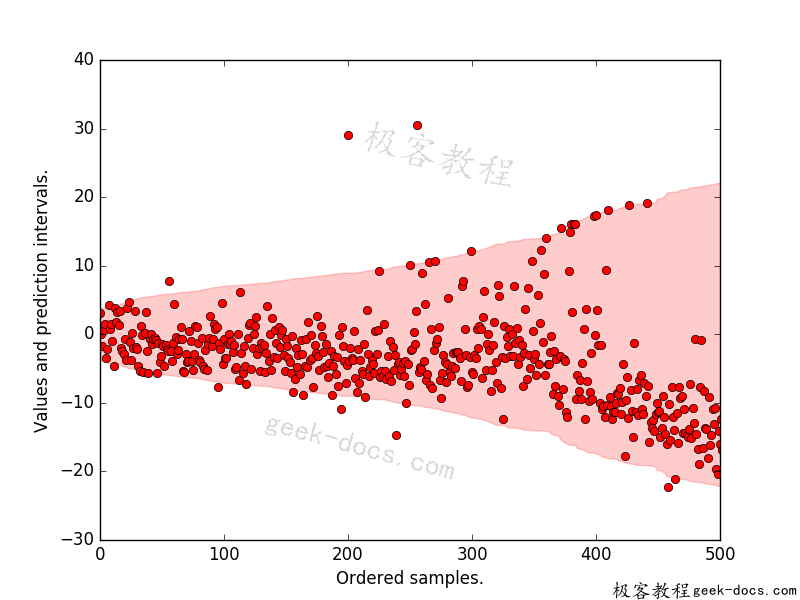# 分位数回归森林

## 分位数回归森林

• 如果它和新样本在同一片叶子上，那么重量就是样本在同一片叶子上的比例。
• 如果没有，则权重为零。

import matplotlib.pyplot as plt
import numpy as np
from sklearn.model_selection import train_test_split
from sklearn.model_selection import KFold
from skgarden import RandomForestQuantileRegressor


boston = load_boston()
X, y = boston.data, boston.target
kf = KFold(n_splits=5, random_state=0)
rfqr = RandomForestQuantileRegressor(
random_state=0, min_samples_split=10, n_estimators=1000)


y_true_all = []
lower = []
upper = []

for train_index, test_index in kf.split(X):
X_train, X_test, y_train, y_test = (
X[train_index], X[test_index], y[train_index], y[test_index])

rfqr.set_params(max_features=X_train.shape // 3)
rfqr.fit(X_train, y_train)
y_true_all = np.concatenate((y_true_all, y_test))
upper = np.concatenate((upper, rfqr.predict(X_test, quantile=98.5)))
lower = np.concatenate((lower, rfqr.predict(X_test, quantile=2.5)))

interval = upper - lower
sort_ind = np.argsort(interval)
y_true_all = y_true_all[sort_ind]
upper = upper[sort_ind]
lower = lower[sort_ind]
mean = (upper + lower) / 2

# Center such that the mean of the prediction interval is at 0.0
y_true_all -= mean
upper -= mean
lower -= mean


plt.plot(y_true_all, "ro")
plt.fill_between(
np.arange(len(upper)), lower, upper, alpha=0.2, color="r",
label="Pred. interval")
plt.xlabel("Ordered samples.")
plt.ylabel("Values and prediction intervals.")
plt.xlim([0, 500])
plt.show()### 评论 1

1. #1

from skgarden import RandomForestQuantileRegressor出现No module named ‘sklearn.ensemble.forest’，请问这是什么原因，怎么解决

so fine1年前 (2020-12-27)回复

• 回顶